# SAT II Math I : Parabolas and Circles

## Example Questions

### Example Question #1 : Parabolas And Circles

Give the axis of symmetry of the parabola of the equation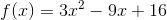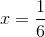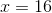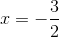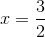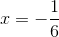Explanation:

The line of symmetry of the parabola of the equation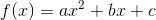is the vertical line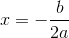Substitute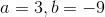:

The line of symmetry is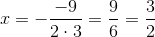That is, the line of the equation.

### Example Question #2 : Parabolas And Circles

What is the center of the circle with the following equation?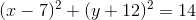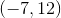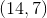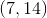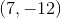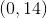Explanation:

Remember that the basic form of the equation of a circle is: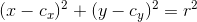This means that the center point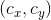is defined by the two values subtracted in the squared terms.  We could rewrite our equation as: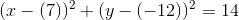Therefore, the center is### Example Question #1 : Parabolas And Circles

What is the area of the sector of the circle formed between the-axis and the point on the circle found at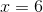when the equation of the circle is as follows?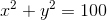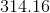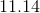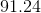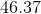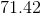Explanation:

For this question, we will need to do three things:

1. Determine the point in question.
2. Use trigonometry to find the area of the angle in question.
3. Use the equation for finding a sector area to finalize our answer.

Let us first solve for the coordinate by substituting into our equation: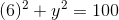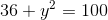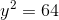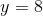Our point is, therefore: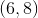Now, we need to calculate the angle formed between the origin and the point that we were given. We can do this using the inverse tangent function. The ratio oftois here: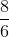Therefore, the angle is: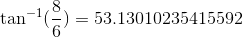To solve for the sector area, we merely need to use our standard geometry equation. Note that the radius of the circle, based on the equation, is.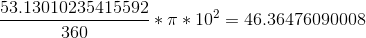This rounds to.

### Example Question #4 : Parabolas And Circles

What is the area of the sector of the circle formed between the-axis and the point on the circle found at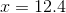when the equation of the circle is as follows?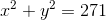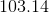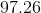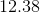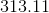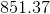Explanation:

For this question, we will need to do three things:

1. Determine the point in question.
2. Use trigonometry to find the area of the angle in question.
3. Use the equation for finding a sector area to finalize our answer.

Let us first solve for the coordinate by substituting into our equation: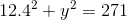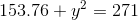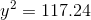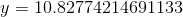Our point is, therefore: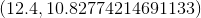Now, we need to calculate the angle formed between the origin and the point that we were given. We can do this using the inverse tangent function. The ratio oftois here: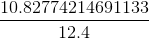Therefore, the angle is: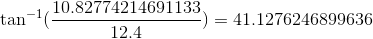To solve for the sector area, we merely need to use our standard geometry equation. Note that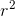, based on the equation, is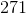.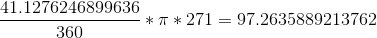This rounds to.

### Example Question #5 : Parabolas And Circles

If the center of a circle is at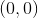and it has a radius of, what positive point on the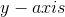does it intersect?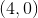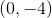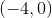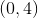Since you are looking for a point on the, yourvalue will be zero.
The center of the circle is at the origin and radius is the distance from the center, so that means the point you are looking for must bepoints away from.
This can be two points on thebut since you are looking for a positive one, your answer must be.